# Excel COUNT and COUNTA functions to count cells

This short tutorial explains the basics of the Excel COUNT and COUNTA functions and shows a few examples of using a count formula in Excel. You will also learn how to use the COUNTIF and COUNTIFS functions to count cells that meet one or more criteria.

As everyone knows, Excel is all about storing and crunching numbers. However, apart from calculating values, you may also need to count cells with values - with any value, or with specific value types. For example, you may want a quick count of all items in a list, or the total of inventory numbers in a selected range.

Microsoft Excel provides a couple of special functions for counting cells: COUNT and COUNTA. Both all very straightforward and easy-to-use. So let's take a quick look at these essential functions first, and then I will show you a few Excel formulas to count cells that meet certain condition(s), and clue you in on the quirks in counting some value types.

## Excel COUNT function - count cells with numbers

You use the COUNT function in Excel to count the number of cells that contain numerical values.

The syntax of the Excel COUNT function is as follows:

COUNT(value1, [value2], …)

Where value1, value2, etc. are cell references or ranges within which you want to count cells with numbers.

In Excel 365 - 2007, the COUNT function accepts up to 255 arguments. In earlier Excel versions, you can supply up to 30 values.

For example, the following formula returns the total number of numeric cells in range A1:A100:

`=COUNT(A1:A100)`

Note. In the internal Excel system, dates are stored as serial numbers and therefore the Excel COUNT function counts dates and times as well.

### Using COUNT function in Excel - things to remember

Below are the two simple rules by which the Excel COUNT function works.

1. If an argument(s) of an Excel Count formula is a cell reference or range, only numbers, dates and times are counted. Blanks cells and cells containing anything but a numeric value are ignored.
2. If you type values directly into the Excel COUNT arguments, the following values are counted: numbers, dates, times, Boolean values of TRUE and FALSE, and text representation of numbers (i.e. a number enclosed in quotation marks like "5").

For example, the following COUNT formula returns 4, because the following values are counted: 1, "2", 1/1/2016, and TRUE.

`=COUNT(1, "apples", "2", 1/1/2016, TRUE)`

### Excel COUNT formula examples

And here are a few more examples of using the COUNT function in Excel on different values.

To count cells with numeric values in one range, use a simple count formula like

`=COUNT(A2:A10)`

The following screenshot demonstrates which types of data are counted and which are ignored:To count several non-contiguous ranges, supply all of them to your Excel COUNT formula. For example, to count cells with numbers in columns B and D, you can use formula similar to this:

`=COUNT(B2:B7, D2:D7)`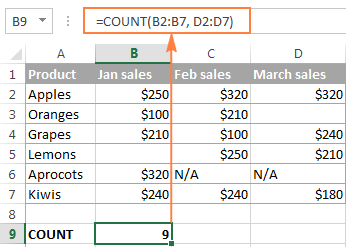Tips:

• If you want to count numbers that meet certain criteria, use either the COUNTIF or COUNTIFS function.
• If apart from numbers, you also want to count cells with text, logical values and errors, use the COUNTA function, which leads us right to the next section of this tutorial.

## Excel COUNTA function - count non-blank cells

The COUNTA function in Excel counts cells containing any value, i.e. cells that are not empty.

The syntax of the Excel COUNTA function is akin to that of COUNT:

COUNTA(value1, [value2], …)

Where value1, value2, etc. are cell references or ranges where you want to count non-blank cells.

For example, to count cells with value in range A1:A100, use the following formula:

`=COUNTA(A1:A100)`

To count non-empty cells in several non-adjacent ranges, use a COUNTA formula similar to this:

`=COUNTA(B2:B10, D2:D20, E2:F10)`

As you can see, the ranges supplied to an Excel COUNTA formula do not necessarily need to be of the same size, i.e. each range may contain a different number of rows and columns.

Please keep in mind that Excel's COUNTA function counts cells containing any type of data, including:

• Numbers
• Dates / times
• Text values
• Boolean values of TRUE and FALSE
• Error values like #VALUE or #N/A
• Empty text strings ("")

In some cases, you may be perplexed by the COUNTA function's result because it differs from what you see with your own eyes. The point is that an Excel COUNTA formula may count cells that visually look empty, but technically they are not. For example, if you accidentally type a space in a cell, that cell will be counted. Or, if a cell contains some formula that returns an empty string, that cell will be counted as well.

In other words, the only cells that the COUNTA function does not count are absolutely empty cells.

The following screenshot demonstrates the difference between Excel COUNT and COUNTA functions: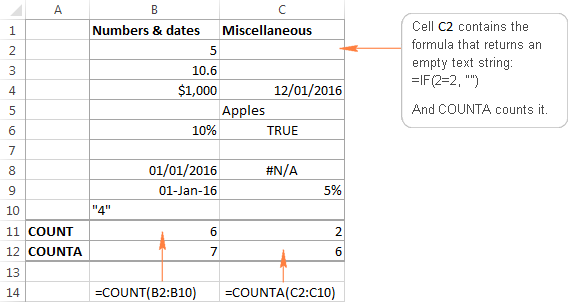For more ways to count non-blank cells in Excel, check out this article.

Tip. If you just want a quick count of non-blank cells in a selected range, simply have a look at Status Bar at the bottom right corner of your Excel window: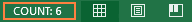## Other ways to count cells in Excel

Aside from COUNT and COUNTA, Microsoft Excel provide a few other functions to count cells. Below you will discuss 3 most common use cases.

### Count cells that meet one condition (COUNTIF)

The COUNTIF function is purposed for counting cells that meet a certain criterion. Its syntax requires 2 arguments, which are self-explanatory:

COUNTIF(range, criteria)

In the first argument, you define a range where you want to count cells. And in the second parameter, you specify a condition that should be met.

For example, to count how many cells in range A2:A15 are "Apples", you use the following COUNTIF formula:

`=COUNTIF(A2:A15, "apples")`

Instead if typing a criterion directly in the formula, you can input a cell reference as demonstrated in the following screenshot: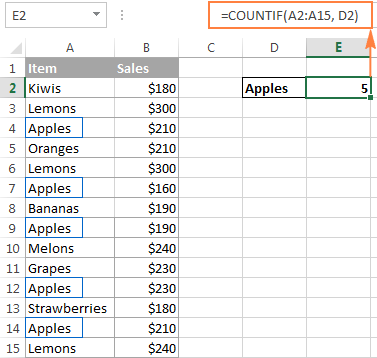### Count cells that match several criteria (COUNTIFS)

The COUNTIFS function is similar to COUNTIF, but it allows specifying multiple ranges and multiple criteria. Its syntax is as follows:

COUNTIFS(criteria_range1, criteria1, [criteria_range2, criteria2]…)

The COUNTIFS function was introduced in Excel 2007 and is available in all later versions of Excel 2010 - 365.

For example, to count how many "Apples" (column A) have made \$200 and more sales (column B), you use the following COUNTIFS formula:

`=COUNTIFS(A2:A15,"apples", B2:B15,">=200")`

To make your COUNTIFS formula more versatile, you can supply cell references as the criteria: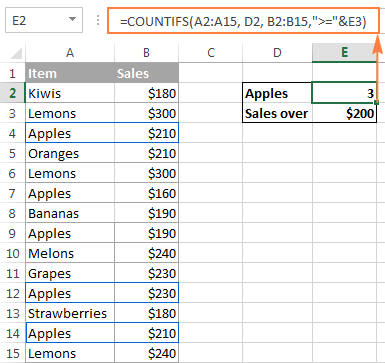You will find plenty more formula examples here: Excel COUNTIFS function with multiple criteria.

### Get a total of cells in a range

If you need to find out the total number of cells in a rectangular range, utilize the ROWS and COLUMNS functions, which return the number of rows and columns in an array, respectively:

`=ROWS(range)*COLUMNS(range)`

For example, to find out how many cells there are in a given range, say A1:D7, use the following formula:

`=ROWS(A1:D7)*COLUMNS(A1:D7)`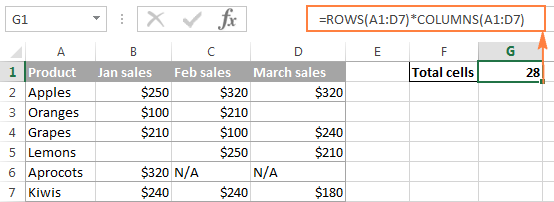Well, this is how you use the Excel COUNT and COUNTA functions. Like I said, they are very straightforward and you are unlikely to run into any difficulty when using your count formula in Excel. If someone knows and is willing to share some interesting tips on to how to count cells in Excel, your comments will be greatly appreciated. I thank you for reading and hope to see you on our blog next week!

## You may also be interested in

1. Hi
I have a excel spreadsheet of members of a club I am running, the spreadsheet contains members details each member has a unique reference number ie, 1243199 etc etc, the members may then have joined more than one group within the club.

I need to be able to show how many members are members of either 1, 2, 3, 4, 5, 6, or 7 groups.

• Simon:
Without having your data in front of me I would say if you select the cells that contain the data you want to analyze and then click Insert/Table you can format the data into a table that you can quickly and easily sort and filter by member or club or whatever information is in the table.
piece of information, go ahead and create headers before you create the table. It makes things much easier to work with.
Depending on the number of members in your club, it might also be easy to create a Pivot Table to analyze this.
Base your Pivot Table on a table and the changes in the table will be reflected automatically in the Pivot Table.
There is a good explanation of Pivot Tables here on AbleBits. Just type "Pivot Table" in the search box and you'll see the link to Pivot Table for Beginners article.

2. HI...
I was tried the formula for sum product
Eg.,
1+2+3+4. this is A Column Values.
I need the Value of Total in B column.
May I know the Formula pls

• Abi:
If I understand your question, where the data in Cells A1:A4 are 1,2,3,4 then in Cell B1 the formula is =Sum(A1:A4)

3. HI...
I was tried the formula for sum product
Eg.,
1+2+3+4. this is A Column Values.
I need the Value of Total is B column.
May I know the Formula pls

4. Hi,
Is there a way to count sequential cells using COUNT A?

I want to have an auto count simply to see if there is data in the boxes 1,3,5,7,9....
and one for 2,4,6,8,10...

Thank you in advance for any help...

5. I am try to write a formula that calculates a range of cells if a second range of cells contains a specific word.

Can you help me in writing this formula.

Thanks

6. If in one cell we will add 14 no e.g. =12+12+15+16+16....than by which formula we can count total entered no ie 14 no??

7. If in one cell we will add 14 no e.g. =12+12+15+16+16....than by which formula we can count total entered now ie 14 no??

8. How to be call apple/oranges in one column..!

9. hi,

10. Hi,
I am trying to find the numbers of people from different districts, I have set all the details of people giving cascading options but in the analysis part, I want the numbers of districts chosen by the data collector, is there any particular formula? so that I can get these numbers of people were from this district and these from another district.
Thanks
Suresh,

11. how to keep attendance daily wise even multiple visit count 1 per day in excel please help me...

Name Date Visit
Ram 2017/12/1 1
Shyam 2017/12/1 1
Hari 2017/12/1 1
Ram 2017/12/1 1
Ram 2017/12/2 1
shyam 2017/12/2 1
Ram 2017/12/31 1
shyam 2017/12/31 1

name month attendance
ram December 3
Shyam December 3
Hari December 1

how to do????

12. Hi,
How to count text mentioned as ''yes'' in different cells Example : count of Yes in cell No. A2 +A20+B15+c30+D25

• Hello,

=COUNTIF(A2,"=yes")+COUNTIF(A20,"=yes")+COUNTIF(B15,"=yes")+COUNTIF(C30,"=yes")+COUNTIF(D25,"=yes")

13. Hello Hi
for exmplale
500-2=498 after one entry amount 498 next new figer enter in cell for
exmpale 3 = 498-3= 495 amount
plese sent me simple formoula

14. Im trying to create a formula that counts yes and no without counting them more than once. The yes can apply to the same person but only need to count it once.

15. Dear,

How to use countifs function in I have sevaral answers in one column that will be counted?

More precisely, if I want to count only "Yes" answers from one column and "Yes, always" and "Yes, sometimes" answers from another.

16. Hello,

2 questions:

1. How to count cells in a row with certain color/s (I use only 2 colors) and return the result in another tab (summary).
2. How to make sure that additional rows in a sheet are counted? I can't highlight the whole column (as range) as I include notes at the bottom of the working area. At the moment, I specify a range but when my list goes beyond the range, and I forget to update the formula, I miss the additional rows and my count is wrong.

Appreciate the help. Thank you.

• Hello, Sarah,

1 Here's a short tutorial about counting cells depending on their colours :)
2 Perhaps, you could extend the range in the formula, but I'm not entirely sure whether you need all the cells from additional range to count or only some of them. And if only some of them, how you decide which ones?

17. Hi, I'm looking for a formula where it only COUNTS a certain criteria in Sheet 2 if it matches my formula in Sheet 1 B2 which is: =COUNTIF(Sheet 2!\$A\$1:\$A\$120,"Dog")

So imagine Sheet 1 B2 having the results already, I now wanted to count either A, B, or C in Sheet 2 if it EXACTLY matches the name I'm looking for in Sheet 1 A1.

e.g: Sheet 1 A1=Dog B1=(count of Dog in Sheet 2) C1=A D1=B E1=C
Sheet 2 A1,A4,A7,A10,A11=Dog
Sheet 2 A2,A5,A8,A12=Cat
Sheet 2 A3,A6,A9,A13=Sheep

Sheet 2 B1=A C1=A D1=A
Sheet 2 B4=B C4=A D4=A
Sheet 2 B7=B C4=B D4=A
Sheet 2 B10=A C10=A D10=A
Sheet 2 B11=A C11=B D11=A

So with this sample given, Sheet 1 B1 counted all "Dog" in Sheet 2. Sheet 1 B1,C1, and D1 is looking for A, B, and C, if all rows in in Sheet 2 says:
[Dog][A][A][A]
[Dog][B][A][A]
[Dog][B][B][A]
[Dog][A][A][A]
[Dog][A][B][A]

Thank you!!!

18. Can someone help me?

I need to Formal for Countif Formal
Ex : Our Employees Duty Roster so Shift Based Job thats reason i Count Different Cell Select then Count How many Employees there Time was There LIke Please Help me

19. Thank you so much for your reply, really appreciate it. I tried the formula but it doesnt give me what am looking for.
This is the summary of the formula i need.
discount of \$37.9/MT for every pound below 49lbs to 47lbs. And discount of \$75.8/MT below 47lbs to 46lbs and rejection for outturn below 46lbs.
Discount of \$5/MT for every nut above 210 to 215, and above 215 to be rejected.
Discount of \$20/MT for every 1% above 10% to 12% above 12% to be rejected. please i need this formula assistance. Thank you.

20. Please i need a formula for a discount of \$37.9 given when a purchase below 49lbs to 47lbs and also a discount of \$75.8 is given when a purchase below 47lbs to 46lbs is been made. please i need a formula fo this please

• Hello.

Thank you for contacting us.

=IF(AND(A1 >= 47,A1 <= 49), "\$ 37.9", IF(AND(A1 >= 46, A1<47), "\$ 75.8", 0))

21. hello !
I need more excel work in today, vlookup and count

22. many number count

23. Can someone help me?

I have a column that has different cities in each cell some have just one city and some have Multiple destinations: city1, city2, city3. These cities overlap. Is there a function I can use to count all of "city 1"? I have tried =COUNTIF(F4:F29,"City 1 name") but it does not count the ones with multiple cities.

Thanks,

24. Could someone please suggest a formula for the following requirement:

For Example: I have in Column range from B5 to B20 and B5, B6, B7, are with numerical data, and B8, B9 are blank, B10,B11 again with data.

I want to have a formula which counts all the cells from B5 to the last active column which is B11 and the answer should be 7 for this – which is by counting all the way from B5 to B11. Many thanks.

• Dear all,

I just got the formula which I was looking for, from another site:

The following formula gives count of all the blank and non-blank, till the last cell with any data:
=MAX(IFERROR(MATCH("ž", A:A),0),IFERROR(MATCH(1E+100, A:A),0))

The following formula give the count of all the blank and non-blank cells till the last cell with the figures
=MATCH(1E+100,A:A)

Hope this would be helpful to someone with the same requirement as mine. God Bless. Thomas

25. I want to count values those are in following ranges
0 -19 ,20-34, 35-39,40-49,50-64,65-74,>75

26. Svetlana - thank you for your blog. Your help on an array three years ago helped me greatly on my project. I have a new COUNTIF question. I have a file with 9895 rows of mailing information. The zip codes are either 5-digits or zip+4. I'm trying to count the number of zip codes in a few categories: 1) Number of 5-digit zip codes (I wrote that formula fine); 2) Number of zip codes that BEGIN with THREE specific numbers and DO NOT include any of the 5-digit zip codes I already counted. I can't seem to write a formula or array that gets me to the correct answer. I don't want to count any zip codes that INCLUDE the three specific numbers if they are NOT the first three numbers. I've used LEFT for a few formulas, but now I can't seem to eliminate those zip codes where the specific string is INSIDE the zip code, not the first three characters in the cell. Should the column of zip codes be a number or text? Here's my array attempt at counting: =SUM((LEN(LABELS!\$B\$2:\$B\$10000)-LEN(SUBSTITUTE(LABELS!\$B\$2:\$B\$9896,"958","")))/LEN("958"))-G2-G3-G4-G7-G8-G9-G10-G11-G12 that I thought searches for zip codes that begin with "958" and subtracts the 5-digit searches I already completed, but it's returning a count with the "958" included in the zip. Any thoughts?

27. Hello
I havew in my column A the fellowing values
B-COM-TES-001
V-MAN-ITF-005
S-COM-TES-020
V-COM-TES-008

if I want to count the combine (COM-TES)In all the column
How can I do

Merci

• =COUNTIF(J4:J7,"*com*")
j4:j7 is data coloumn.

• I have date in cell like below:
TC1D 2
TC1-D 5
I want to count the date cell for both at a time and sum of the same.
In that way like my count will come 2 and sum 7.

28. Hello,

Do you also have a tutorial on COUNT and COUNTA in Google Sheets?

Yours,

Robert

29. Hi there,

I want to count the number of blank cells and non-blank cells in column B,
if column A is X and column C is X, Y and Z.

Thank you

30. I have data with family includes Self, spouse, children, parents. i want separate count of how many single child family and how may 2 children family are there in data.

kindly help

31. Hi,

I would love to have some help on this:

I have B5:B66 range and I want count ONLY if a certain value occurs 3 times or more (values 8,0

Example: 8599962555
I need to count: 999 = 1
and: 555 = 1
Then : 1+1 = 2

How can I do this???

33. Hi, i have a certain name (wilium) in column A and against this name i have 2 option, 1 Done and 2 Inprogress. i want that i count done again wilium and count inprogress against wilium separately. which formula will work for it??

34. how can I make formulas appear in all cells instead of the values

• ctrl+`

35. I have query divide this no as an following way in different column if 4,50,00, 000 given then we will get
2,00,00,000
1,00,00,000
1,00,00,000
50,00,000

36. Ok guys/girls i'm pulling my hair out with this formula, i'm fairly confident the problem is in the two cells with times in. cells h50:h5000 have two times combined in a cell with - as a division, all i need is the second part but using 'right' function doesn't appear to work as part of a larger countifs. these cells are imported and can be 1000's of rows long so creating another column to separate is awkward.

f50:f5000=13:59 h50:h5000=12:33-17:00

=COUNTIFS(C\$50:C\$5000,A6, X\$50:X\$5000, ">0", Y\$50:Y\$5000, ">0",F\$50:F\$5000, ">" & RIGHT(H\$50:H\$5000,5))

The formula by itself (ctrl, shift, enter) appears to work fine but I require the other criteria.

=COUNTIF(F\$50:F\$5000, ">" & RIGHT(H\$50:H\$5000,5))

Any help would be greatly appreciated.

Andy

37. Hi,
I am trying to use countifs to count no of cell which meet multiple criteria where each criteria has got different no fo rows and column compare to other.
for example:
i want to count no of cell from column D,E and F if value in column A is equal to X.

38. Hi Mam
This list is % or marks
100
93
91.4
90.8
90.6
90
88.6
86.4
85.2
80.8
79.2
78.4
75.8
75
73.6
73.6
73.6
73.6
73.6
73.6
73.6
73.6
71.6
71.6
71.4
70
69.6
68.6
66.2
65.2
I want result between 91 to 100 how many student
81 to 90
71 to 80
61 to 70
51 to 60
41 to 50
33 to 40
below 33
what formula use in it.

• =countifs(range of mark,">=71",range of mark,"<=80")

39. Hello I am trying to find a formula which allows me to add 2 different words in 2 different columns with drop down menus in each. Here are an example of the words:

"A" for Column E

For Column G
ACV, AMV, FIRE, PER INJ, OTHER, PMV2, PMV4, PMVO, PRO DMG, SUPLMT, WATER, WPN/ND, AV GND

A certain combination will only work for a specific column. Example:
Accident Class:
A
B
C
D
E
F
OHR
NR
Each of these only work with one word at a time, but all of the combinations for "A" need to be tracked in a column (A3) for example.
"B" would tracked in A4 and "C" would be tracked in A5 and so on. If B and ACV were in their respective columns (Say E5 and G5)on one sheet, then those 2 numbers would be tracked on another sheet on A3 as a number.

I hope this explains it well enough, thank you.

• I found the solution, disregard, thank you.

40. I am using this formula =IF(MAX(\$A\$4:A263)=SUMPRODUCT(1/COUNTIF(\$B\$5:B264,\$B\$5:B264)),"",(SUMPRODUCT(1/COUNTIF(\$B\$5:B264,\$B\$5:B264)))) For Serial Number but it shows the sr no after missed some columns. whereas the sr. number column should be nill until the next particular not comes.

41. I want to count enteries in a column and then multiple the number of entries by a set number. In other words, I have a spreadsheet, where different columns have different values. IF an entry is made in column A, it should be counted as 1 entry. But the value of second column is double and that of third is x 5.

So I want to be able to count the number of entries in a particular column and then multiply it by 2/5/10. Whats the best formula for this?

42. Write a Formula to count the number of Active Employees in Each City

Employees Location Status
Employee1 Manila Active
Employee2 Manila Active
Employee3 Mumbai Active
Employee4 Surrey Active
Employee5 Mumbai Inactive
Employee6 Mumbai Active
Employee7 Mumbai Active
Employee8 Manila Inactive
Employee9 Manila Active
Employee10 Surrey Inactive
Employee11 Surrey Active
Employee12 Surrey Active
Employee13 Manila Active
Employee14 Manila Active
Employee15 Manila Active
Employee16 Manila Active
Employee17 Manila Inactive
Employee18 Surrey Active
Employee19 Surrey Active
Employee20 Mumbai Active

43. HOW TO COUNT, I HAVE DATA OF STUDENTS FROM VARIOUS STATES, I WANT TO COUNT HOW MANY STUDENTS ARE FROM DELHI AND THERE SURNAME IS "REDDY" ? PLZ HELP

• =COUNTIFS(G2:G11,"****reddy",H2:H11,"delhi")

44. I have want to count a range of cells. The =COUNTA('Employee Edu-Skills History'!A31:A55,"*")count the formulas. I only want to count the text in the cells

45. Hello

i want to count Yes and No in the rows

That was no issue .
But i want to stop the you after the first the NO

has sombody a idea how to handle it ?

46. I was Searching this topic since 3 weeks Now i Found in this articular Thanks a lot. its working in Drop Down list active Words.
=COUNTIF(A2:A15, "apples")

47. I have three worksheets two with the years dates in B3:NB3. the a1 column has text in it. I am trying to calculate how many time in a rolling 90 days the product in a1 sell mp I'm using this
=COUNTIFS(A1,"<=today()-90",'2016'!B4:NB4,"MP")

It count the MP but
ie if today is 5/24/16 it should not count any mp before 2/27/16

can you tell me what I'm missing. I'm getting "Value" in the output field

thanks

48. can u send me a drop down list examples in excel where there is huge data for e.g

Divison Zone Region Area HQ
Divan West Mumbai Mumbai Mumbai
Divan West Mumbai Mumbai Goa
Divan West Pune Pune Pune
Divan West Pune Pune Pimpri
Divan West Ahmdabd Rajkot Rajkot
Siman North Delhi dELHI Delhi
Siman North Delhi jAIPUR Kota
Siman North Delhi Jaipur Jodhpur

if we click on Division the Divison will show then after clicking on Zone the zone and clicking on the specific zone the zones area will reflect with HQ automatically.

• Hi Rohan,

Please check out the following tutorials:

49. Pretty good ma'm, bundle of thanks :)

50. how can we use "if" function when there is either text or number in cell..

if the cell contain text then answer should be "Yes" or else the cell contain number then answer shold come "No"

• Hello Rinju,

You can use a formula similar to this:

=IF(ISTEXT(A1), "Yes", IF(ISNUMBER(A1), "No", ""))

Additionally, you can check for blank cells:

=IF(ISTEXT(A1),"yes", IF(ISNUMBER(A1),"no", IF(ISBLANK(A1),"blank","")))